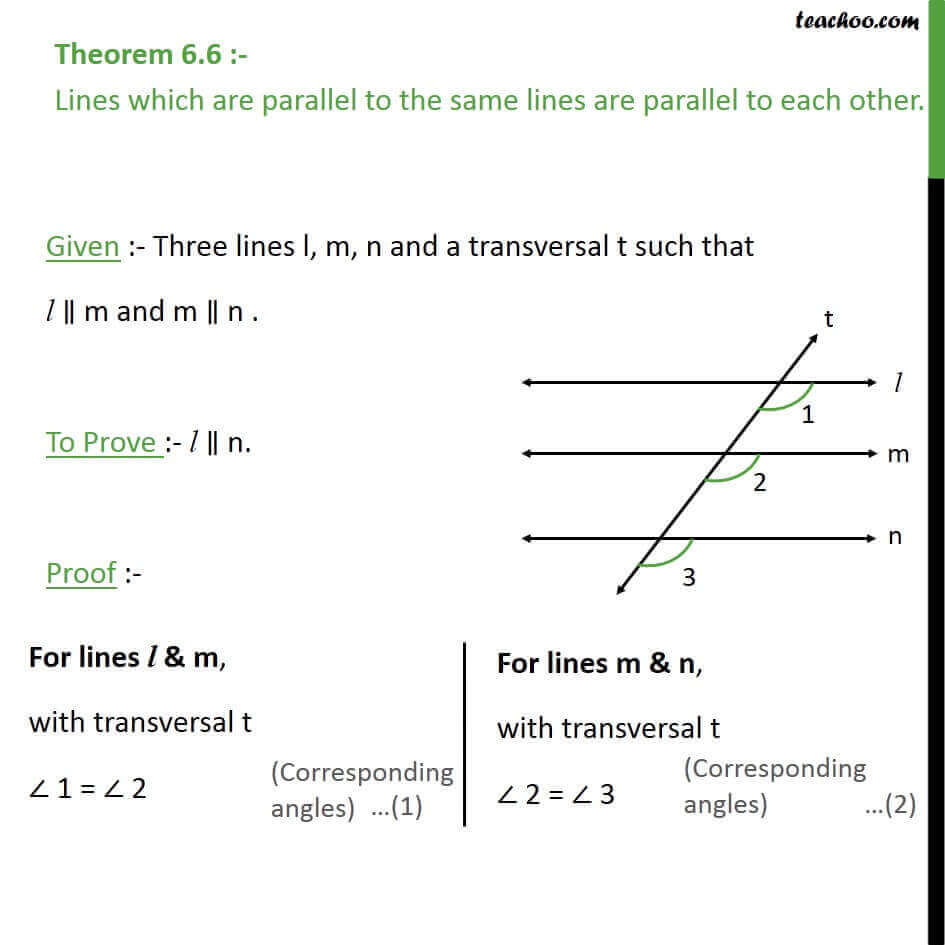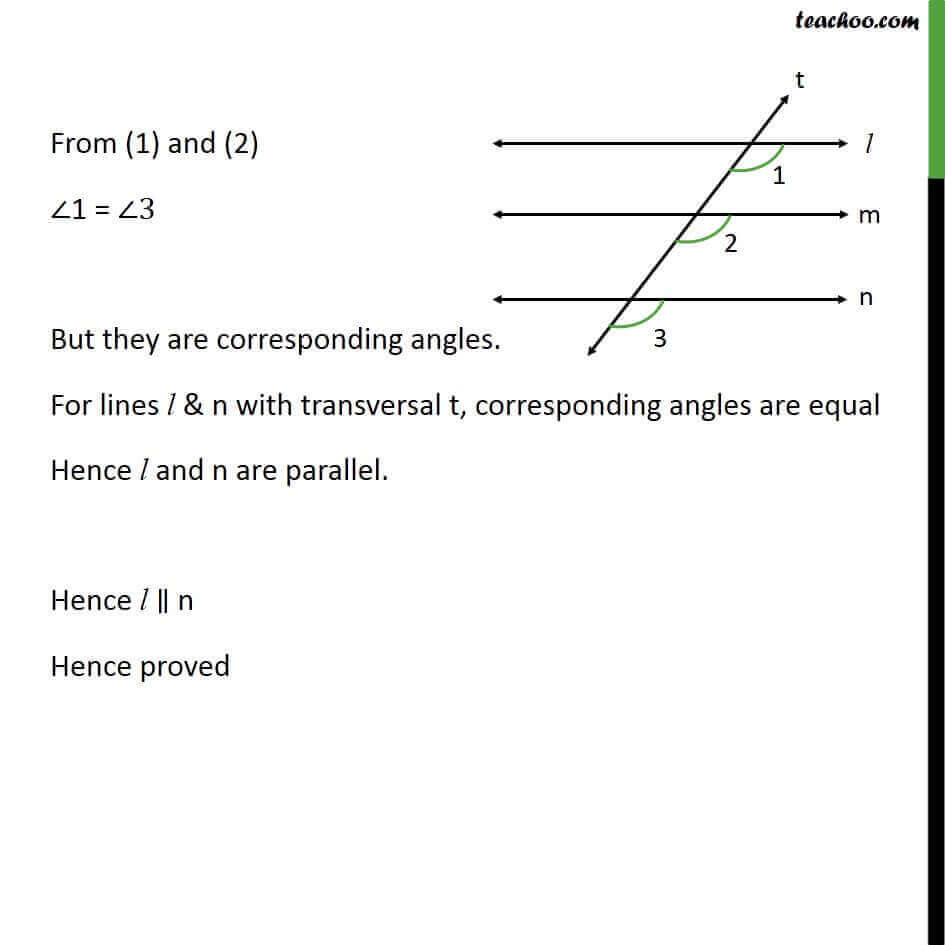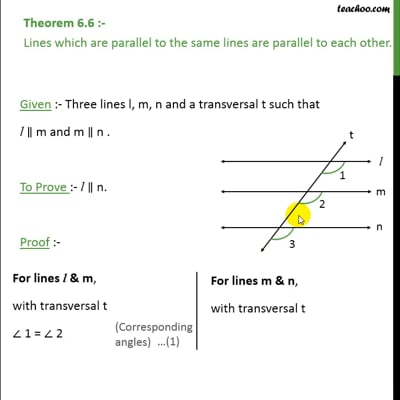Theorems

Chapter 6 Class 9 Lines and Angles (Term 1)
Serial order wiseThis video is only available for Teachoo black users

### Transcript

Theorem 6.6 :- Lines which are parallel to the same lines are parallel to each other. Given :- Three lines l, m, n and a transversal t such that l m and m n . To Prove :- l n. Proof :- From (1) and (2) 1 = 3 But they are corresponding angles. For lines l & n with transversal t, corresponding angles are equal Hence l and n are parallel. Hence l n Hence proved